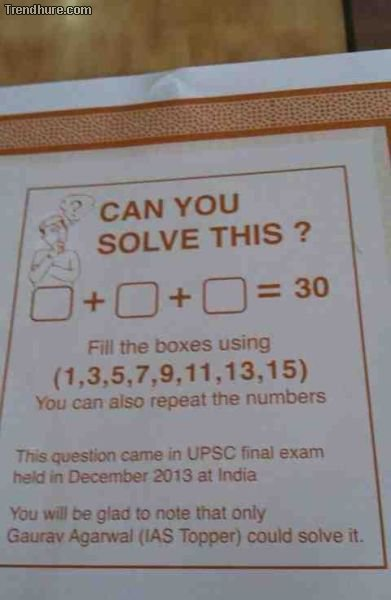Today i found this picture on the internetz:Basically you have to find a solution for a + b + c = 30, where a, b and c can be one of 1, 3, 5, 7, 9, 11, 13, 15 (with repetition).

After try some things on a paper with drawing trees, I felt that there is probably no solution at all for this exercise. So I thought I should use some brute force to figure out a solution - and python comes in handy here:

``````import itertools

items = [1,3,5,7,9,11,13,15]
r = 3
target = 30

for test in itertools.combinations_with_replacement(items, r):
if sum(test) == target:
print(test)
``````

Well. No solution with this brute force attack... I would say the exercise is a joke. Interessting question: How can you proove that it is a joke? The brute force attack is basically a proof by exhaustion. I thought there is probably some primes involved...

EDIT: OK this might be a proof that such a question is not solveable: All Numbers are odd. If you add two odd number, you get an even number. If you add an even number to an odd number, you will end up on an odd number. So for an odd number of numbers to be added up, the result will be odd as well. 30 is even, so there is no solution to this equation given only odd numbers.